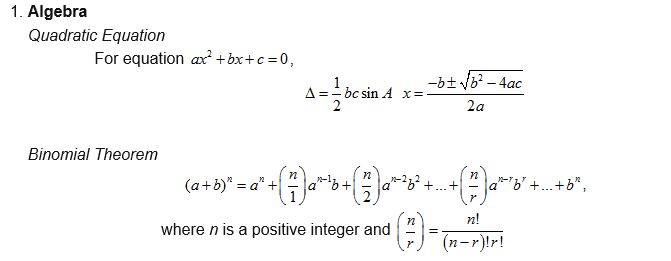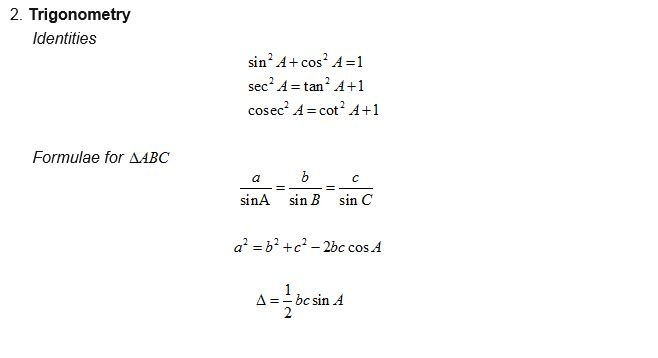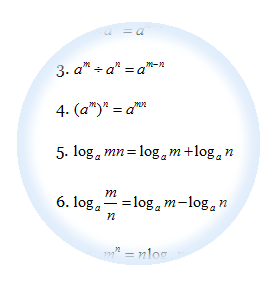# The Key to Math Success: Why Home Tuition Matters

Mathematics is a subject that both fascinates and challenges students of all ages. For parents, ensuring their child’s success in math is often a top priority. Home tuition is a valuable resource that can make a significant difference in a child’s mathematical journey. In this article, we’ll explore the key topics parents care about when it comes to home tuition for math.

Continue reading The Key to Math Success: Why Home Tuition Matters

# Formulae that will be given in IGCSE 0606 Additional Mathematic

These are the formulae that will be given in the IGCSE 0606 Additional Maths Exam to help you to answer the Questions. Basically there are divided into 2 categories:# Indices and Logarithms

Indices$\text{Positive Index: }{{a}^{n}},a\ne 0$$eg:{{2}^{3}},{{4}^{2}}$$\text{Negative Index: }{{a}^{-n}}=\frac{1}{{{a}^{n}}}$$eg:{{2}^{-3}}=\frac{1}{{{2}^{3}}}=\frac{1}{8}$$\text{Zero Index: }{{a}^{0}}=1,a\ne 0$$eg:{{2}^{0}}=1$

Fractional Indices:${{a}^{\frac{1}{n}}}=\sqrt[n]{a},a\ne 0$$eg:{{8}^{\frac{1}{3}}}=\sqrt{8}=2$${{a}^{\frac{m}{n}}}={{(\sqrt[n]{a})}^{m}},a\ne 0$$eg:{{8}^{\frac{2}{3}}}={{(\sqrt{8})}^{2}}={{(2)}^{2}}=4$

Law of Indices:$\text{Rule 1: }{{a}^{m}}\times {{a}^{n}}={{a}^{m+n}}$$eg:{{2}^{2}}\times {{2}^{3}}={{2}^{2+3}}={{2}^{5}}=32$$\text{Rule 2: }{{a}^{m}}\div {{a}^{n}}={{a}^{m-n}}$$eg:{{2}^{4}}\div {{2}^{2}}={{2}^{4-2}}={{2}^{2}}=4$$\text{Rule 3: }{{({{a}^{m}})}^{n}}={{a}^{m\times n}}$$eg:{{({{2}^{2}})}^{3}}={{2}^{2\times 3}}={{2}^{6}}=64$

# Formulae that will be given in SPM Add MathsThese are the formulae that will be given to help you to answer the SPM Additional Maths Questions. However, more important is that you know how to apply those formulaes to solve your questions. Buy some exercises from the bookstore and work on the questions everyday and you will master all those formulaes. Basically there are divided into 5 categories:

1. Algebra
2. Calculus
3. Statistics
4. Geometry
5. Trigonometry

# SPM Questions for Simultaneous Equations

At least one simultaneous equations question (5 marks) will be coming out in the SPM paper 2, section A.

1. Substitution (SPM 2005, SPM2007)
2. Using Formulae (SPM 2006, SPM 2009)

# SPM Questions for Quadratic Functions

1. The Basic of quadratic functions (SPM 2010)
2. Determine max and min values of quadratic function (SPM2009)
3. How to sketch the graph of quadratic functions (SPM2010)
4. How to find the range of values of x in Quadratic inequalities (2007, 2006)This topic is closely related to the topic of quadratic equations. We will discuss further  on 4 subtopics below:

1. The Basic of quadratic functions
2. Determine max and min values of quadratic function
3. How to sketch the graph of quadratic functions
4. How to find the range of values of x in Quadratic inequalities

# SPM Questions for Quadratic Equations

We will discuss some pass year SPM exam questions based on:

• Solve the quadratic equations – SPM 2003, SPM 2005
• Form a quadratic equation – SPM 2009
• Determine the conditions for the type of roots – SPM2010

## 3 Basic Techniques in Solving Quadratic Equation Questions

In this chapter we will learn 3 most basic techniques on how to: